Custom SearchDISCONTINUITIES The discussion of discontinuties will be based on a comparison to continuity. A function, f(x), is continuous at x = a if the following three conditions are met: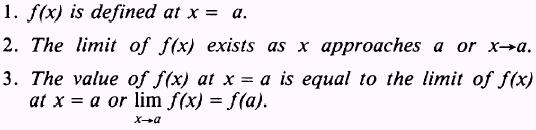If a function f(x) is not continuous at x=a then it is said to be discontinuous at x=a We will use examples to show the above statements. EXAMPLE: In figure 4-4, is the function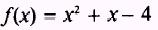SOLUTION: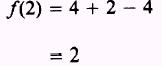and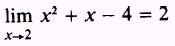andFigure 4-4.-Function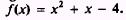Therefore, the curve is continuous at x=2 EXAMPLE: In figure 4-5, is the functioncontinuous at f(2)? SOLUTION: f(2) is undefined at x=2 and the function is therefore discontinuous at x=2Figure 4-5.-FunctionHowever, by extending the original equation of f(x) to readwe will have a continuous function at The value of 4 at x = 2 was found by factoring the numerator of f(x) and then simplifying. A common kind of discontinuity occurs when we are dealing with the tangent function of an angle. Figure 4-6 is the graph of the tangent as the angle varies from 0 to 90; that is, from 0 to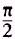. The value of the tangent atis undefined. Thus the function is said to be discontinuous at 2.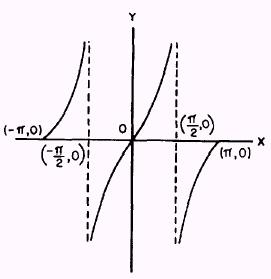Figure 4-6.-Graph of tangent function. PRACTICE PROBLEMS: In the following definitions of the functions, find where the functions are discontinuous and then extend the definitions so that the functions are continuous:ANSWERS: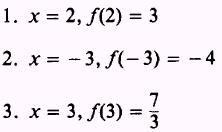Integrated Publishing, Inc. - A (SDVOSB) Service Disabled Veteran Owned Small Business